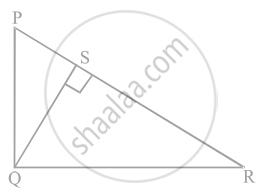# In figure, PQR is a right triangle right angled at Q and QS ⊥ PR. If PQ = 6 cm and PS = 4 cm, find QS, RS and QR. - Mathematics

Sum

In figure, PQR is a right triangle right angled at Q and QS ⊥ PR. If PQ = 6 cm and PS = 4 cm, find QS, RS and QR.#### Solution

Given, ΔPQR in which ∠Q = 90°, QS ⊥ PR and PQ = 6 cm, PS = 4 cm

In ΔSQP and ΔSRQ,

∠PSQ = ∠RSQ  .....[Each equal to 90°]

∠SPQ = ∠SQR   .....[Each equal to 90° – ∠R]

∴ ∠SQP ∼ ∠SRQ

Then, (SQ)/(PS) = (SR)/(SQ)

⇒ SQ^2 = PS xx SR   .....(i)\

In right angled ΔPSQ,

PQ^2 = PS^2 + QS^2   ......[By Pythagoras theorem]

⇒ (6)^2 = (4)^2 + QS^2

⇒ 36 = 16 + QS^2

⇒ QS^2 = 36 - 16 = 20

∴ QS = sqrt(20) = 2sqrt(5) cm

On putting the value of QS in equation (i), we get

(2sqrt(5))^2 = 4 xx SR

⇒ SR = (4 xx 5)/4 = 5 cm

In right angled ΔQSR,

QR^2 = QS^2 + SR^2

⇒ QR^2 = (2sqrt(5))^2 + (5)^2

⇒ QR^2 = 20 + 25

∴ QR = sqrt(45) = 3sqrt(5) cm

Hence, QS = 2sqrt(5) cm, RS = 5 cm and QR = 3sqrt(5)` cm

Concept: Application of Pythagoras Theorem in Acute Angle and Obtuse Angle
Is there an error in this question or solution?

#### APPEARS IN

NCERT Mathematics Exemplar Class 10
Chapter 6 Triangles
Exercise 6.4 | Q 10 | Page 74

Share